Learn All Concepts of Chapter 2 Class 11 Relations and Function - FREE. Check - Relation and Function Class 11 - All Concepts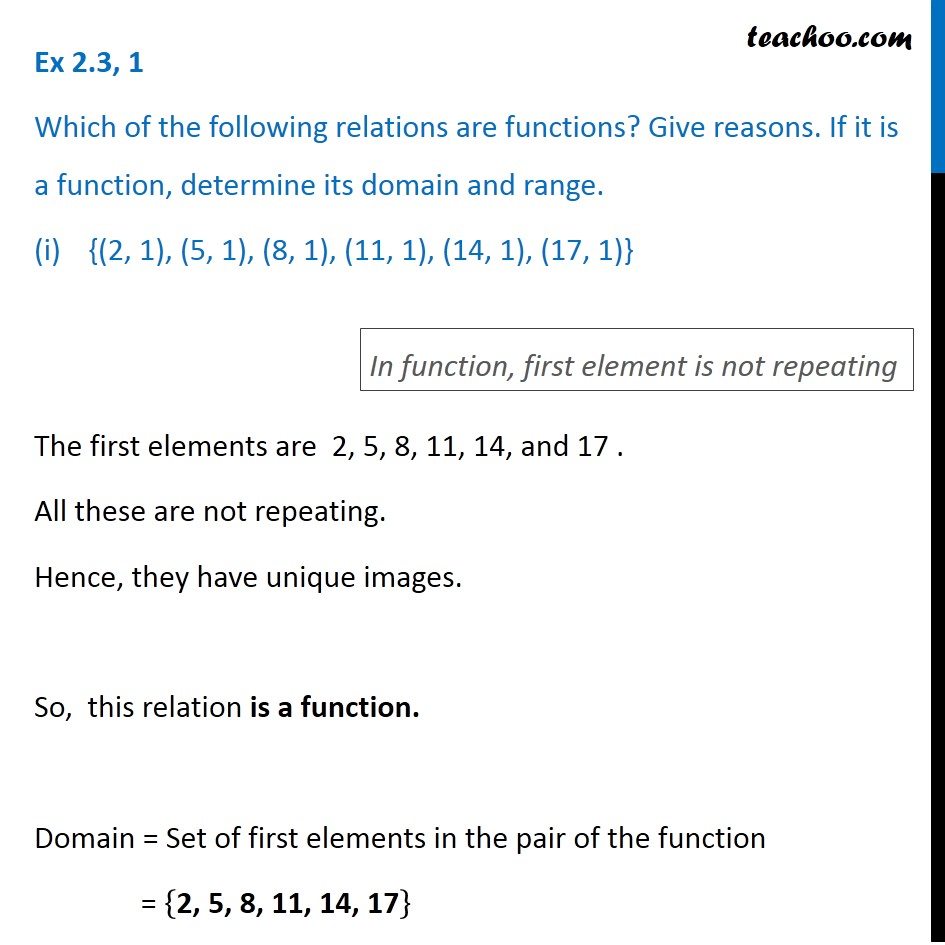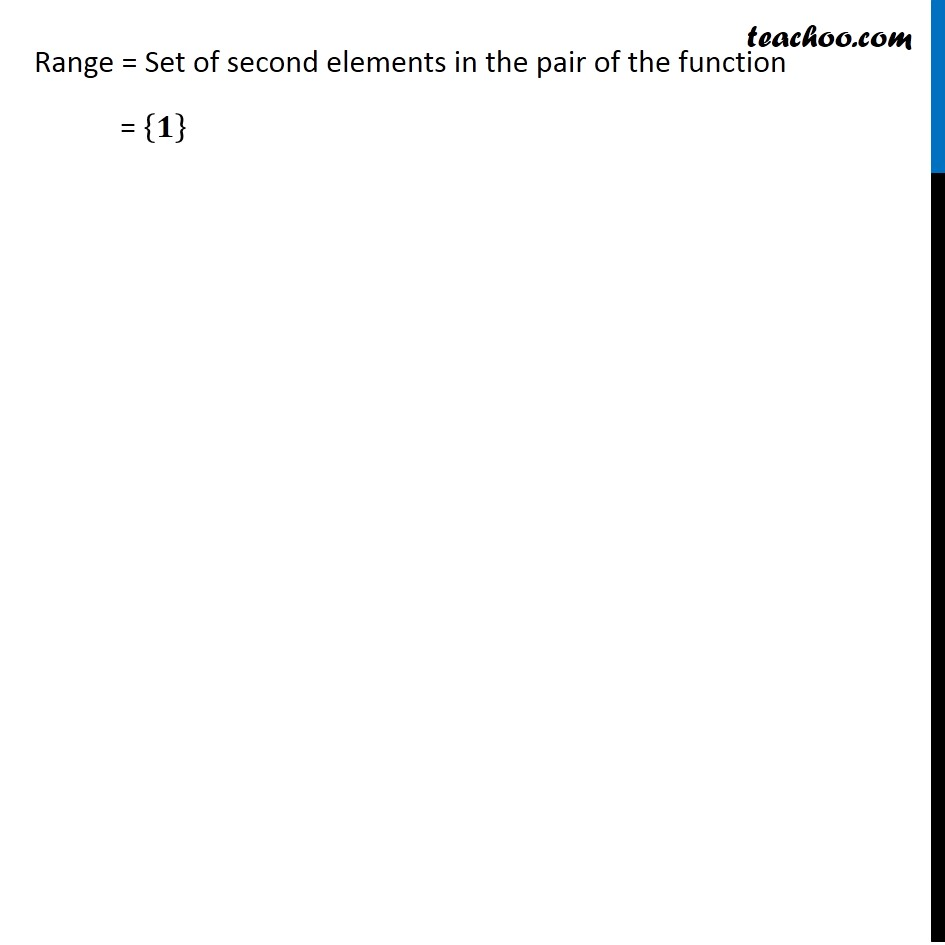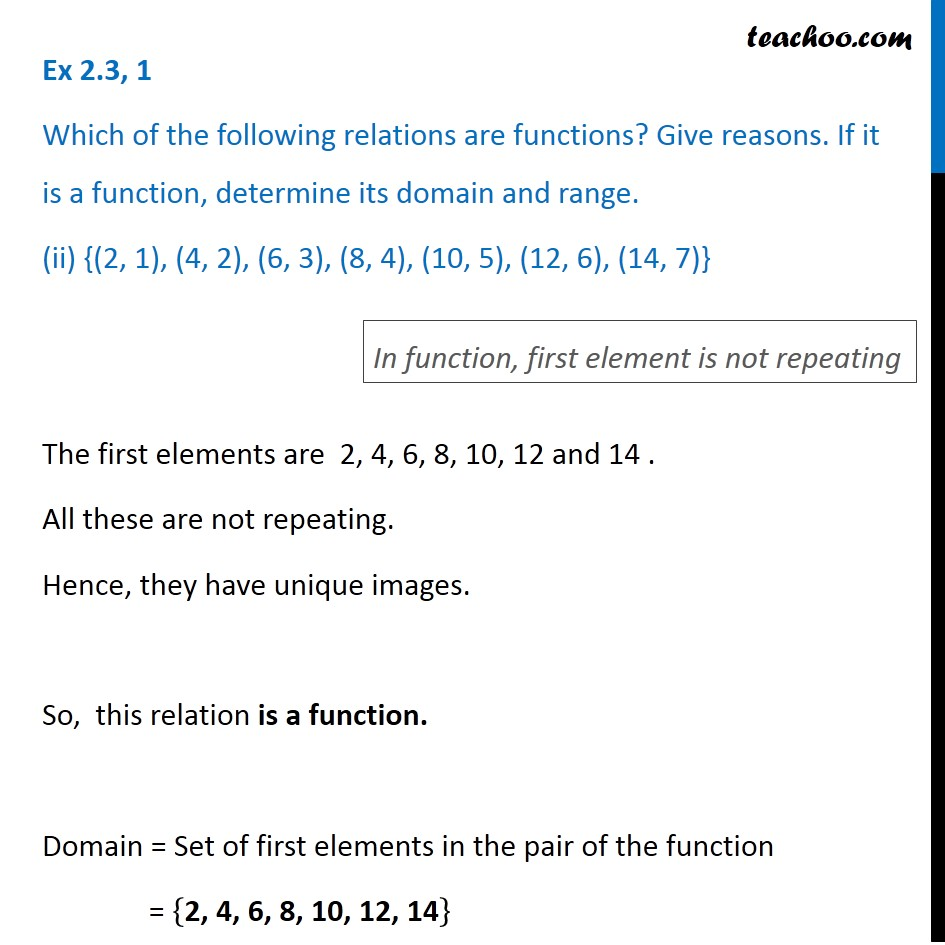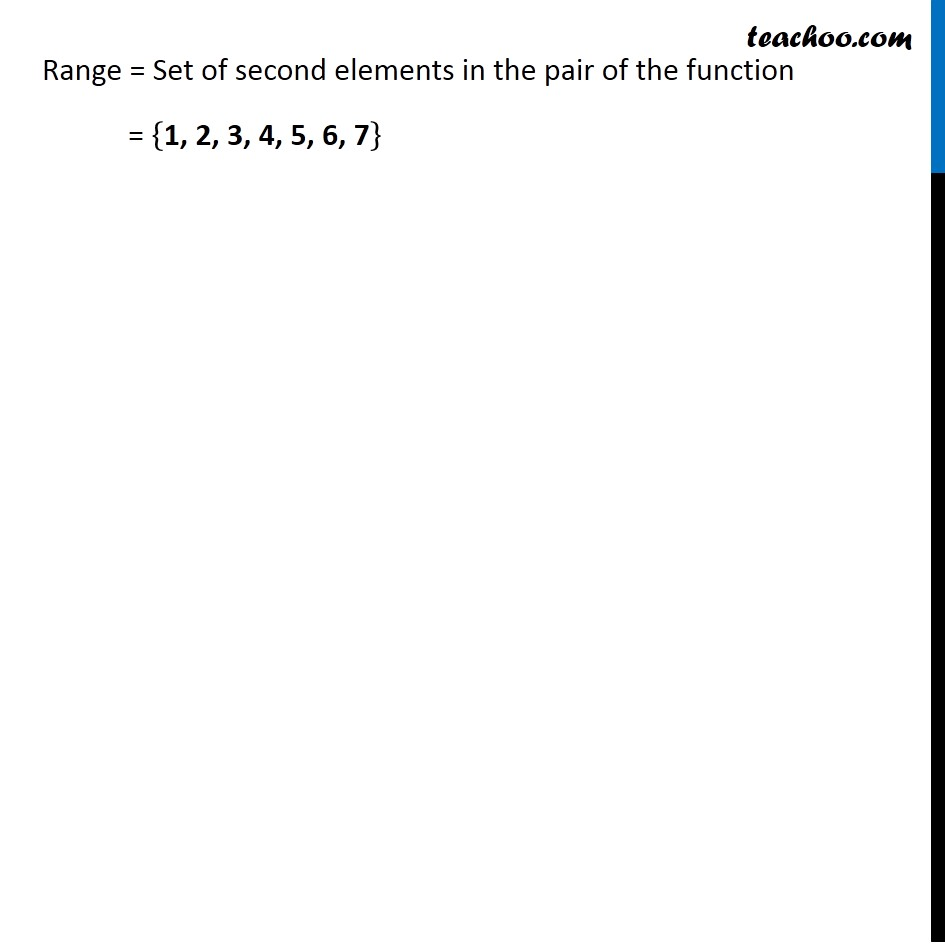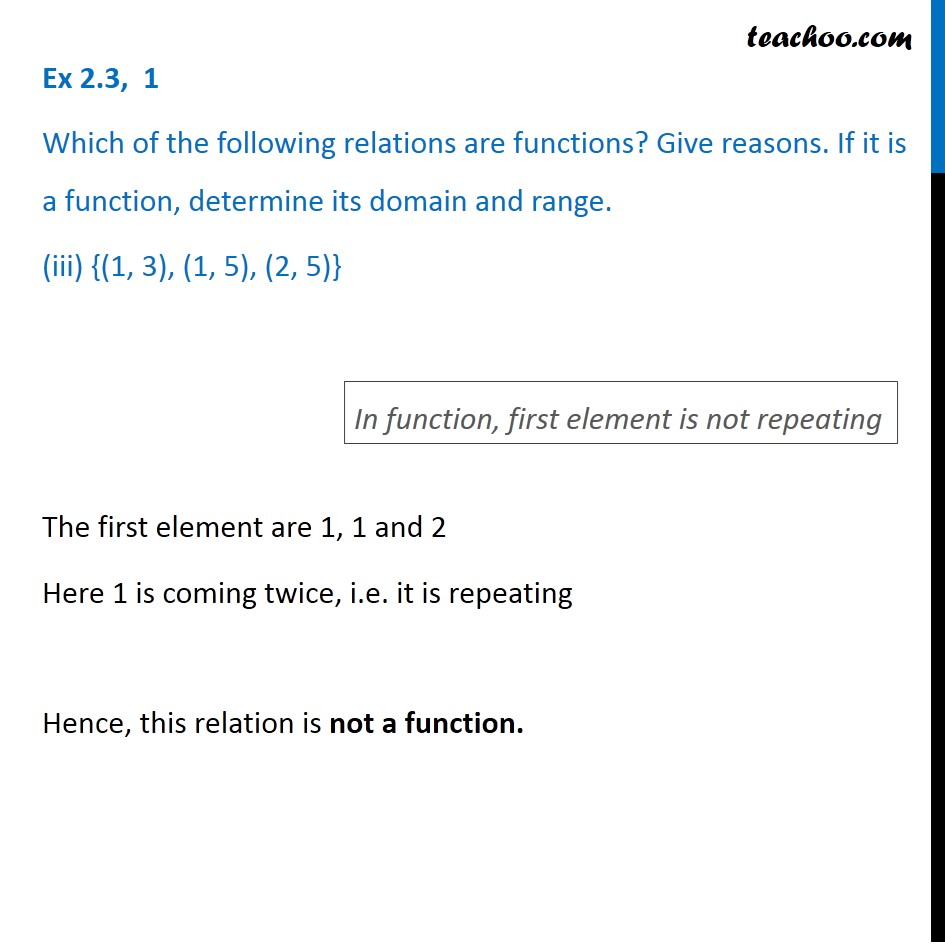1. Chapter 2 Class 11 Relations and Functions
2. Serial order wise
3. Ex 2.3

Transcript

Ex 2.3, 1 Which of the following relations are functions? Give reasons. If it is a function, determine its domain and range. {(2, 1), (5, 1), (8, 1), (11, 1), (14, 1), (17, 1)} The first elements are 2, 5, 8, 11, 14, and 17 . All these are not repeating. Hence, they have unique images. So, this relation is a function. Domain = Set of first elements in the pair of the function = {"2, 5, 8, 11, 14, 17" } In function, first element is not repeating Range = Set of second elements in the pair of the function = {𝟏} Ex 2.3, 1 Which of the following relations are functions? Give reasons. If it is a function, determine its domain and range. (ii) {(2, 1), (4, 2), (6, 3), (8, 4), (10, 5), (12, 6), (14, 7)} The first elements are 2, 4, 6, 8, 10, 12 and 14 . All these are not repeating. Hence, they have unique images. So, this relation is a function. Domain = Set of first elements in the pair of the function = {"2, 4, 6, 8, 10, 12, 14" } In function, first element is not repeating Range = Set of second elements in the pair of the function = {"1, 2, 3, 4, 5, 6, 7" } Ex 2.3, 1 Which of the following relations are functions? Give reasons. If it is a function, determine its domain and range. (iii) {(1, 3), (1, 5), (2, 5)} The first element are 1, 1 and 2 Here 1 is coming twice, i.e. it is repeating Hence, this relation is not a function. In function, first element is not repeating

Ex 2.3